matlab做图像处理_精通matlab数字图像处理与识别 matlab - CSDN
• 1.1 MATLAB图像处理基本操作 本文中对于大多数的操作，是对数字图像处理领域中最为著名的“lena”图片进行操作的。原图如下（Figure 1）：  Figure 1 首先，在MATLAB中显示这幅图片： I=imread('lena.jpg')...
1.1  MATLAB图像处理基本操作

本文中对于大多数的操作，是对数字图像处理领域中最为著名的“lena”图片进行操作的。原图如下（Figure 1）：Figure 1

首先，在MATLAB中显示这幅图片：

I=imread('lena.jpg');
imfinfo('lena.jpg')
imshow(I);Figure 2

从Figure 2中可以看出，该图片格式为png，长宽均为512px，颜色类型为真彩色。

处理后的图片还需要进行保存：

imwrite(I,'lenaSave.jpg');

1.2  图像数字化

为了完成这样一个步骤，MATLAB中提供了相应的函数：

I1=rgb2gray(I);
imshow(I1);

I1以512*512*1的矩阵形式进行存储。效果如下图（Figure 3）：Figure 3

1.3    直方图

为了显示图像灰度的分布情况，还需要绘制灰度直方图。可以使用如下代码：

figure,imhist(I1);%显示图片灰度值的统计结果直方图

效果如下（Figure 4）：Figure 4

1.4   图像噪声的添加

为了完成多种图像处理的操作和试验，还可以对图片添加噪声。所用函数为imnoise (I, type)，该函数中的type可以为5种噪声参数，分别为：'gaussian'(高斯白噪声)，'localvar'(与图象灰度值有关的零均值高斯白噪声)，'poisson'(泊松噪声)，'salt & pepper'(椒盐噪声)和'speckle'(斑点噪声)。

以下为高斯噪声（Figure 5）和椒盐噪声（Figure 6）的代码和效果：

I2=imnoise(I1,'gaussian');
figure,imshow(I2);Figure 5

I3=imnoise(I1,'salt & pepper');
figure,imshow(I3);Figure 6


展开全文• 图像增强中两类重要的处理方法：一种是灰度变换，另一种是直方图处理。  一、灰度变换  灰度变换通常可分为线性变换、分段线性变换和非线性变换。我们将分别给出实验，来查看其处理效果。  1、线性变换 ...

图像增强中两类重要的处理方法：一种是灰度变换，另一种是直方图处理。

一、灰度变换

灰度变换通常可分为线性变换、分段线性变换和非线性变换。我们将分别给出实验，来查看其处理效果。

1、线性变换

例1：我们对一张较暗的图片进行简单的加法，提高他的亮度，从而可以看到隐藏在黑暗中的细节。

代码：

figure,imshow(I);

title('bright_dark.jpg');

J=I+100;

figure,imshow(J);

title('给每个像素增加100');

效果图：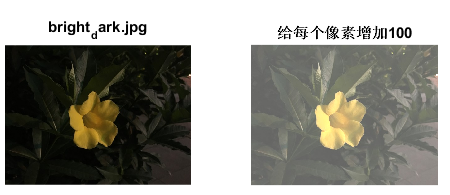2、分段线性变换

例2：下面是分段线性变换的算法及变换前后的对比图像。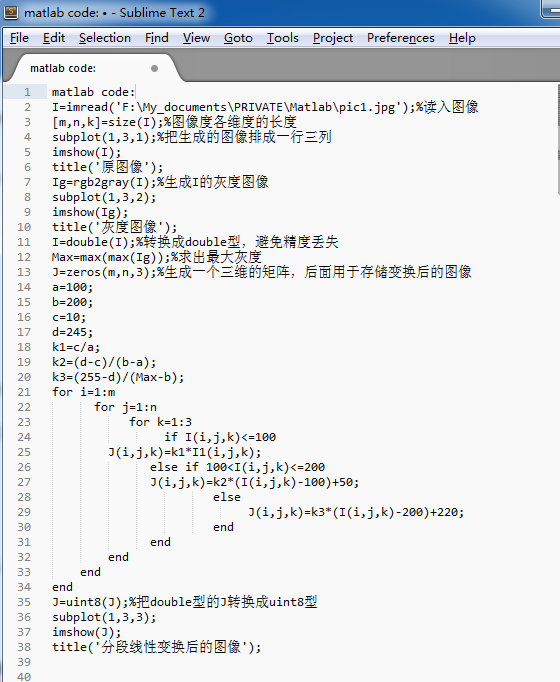对比图像：3、非线性变换

例3：下面是一个对数变换的算法和实验效果图。

I=mat2gray(I);   %对数变换不支持uint8类型的数据，将一个矩阵归一化为灰度图像的数据格式

J=log(I+1);

subplot(1,2,1);

imshow(I);

title('原图像');

subplot(1,2,2);

imshow(J);

title('对数变换后的图像');

效果图：4、还有一种比较常用的线性变换方法——图像取反

取反操作适于增强嵌入暗色区域的白色或灰色细节，当整幅图像偏暗时，效果尤为明显。

例3：

subplot(1,2,1);

imshow(I);

title('原图像');

J=imcomplement(I);

subplot(1,2,2);

imshow(J);

title('取反后的图像');

效果对比：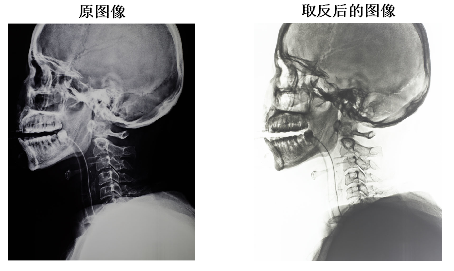相同的效果可由以下代码实现。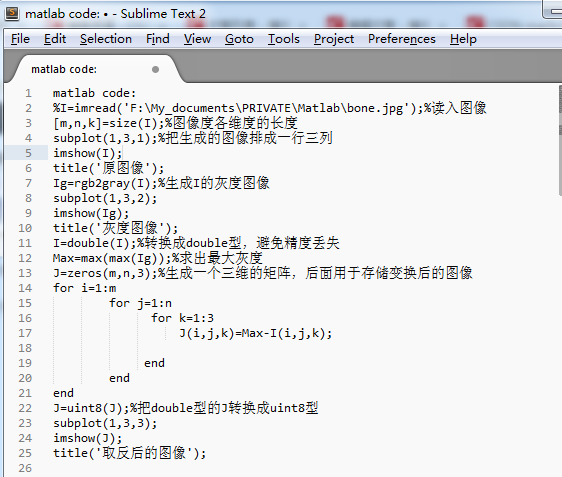灰度变换可使图像动态范围增大，对比度得到扩展，使图像清晰、特征明显，是图像增强的重要手段之一。它主要利用点 运算来修正像素灰度，由输入像素点的灰度值确定相应输出点的灰度值，是一种基于图像变换的操作。灰度变换不改变图像内 的空间关系，除了灰度级的改变是根据某种特定的灰度变换函数进行之外，可以看作是“从像素到像素”的复制操作。

二、直方图变换

图像的灰度统计直方图是一种函数，它表示数字图像中每一个灰度级与该灰度级出现的频数的关系。图像的直方图显示了图像的外貌，暗的图像，其直方图的组成部分集中在灰度较低的一侧；明亮的图像，其直方图的组成部分则集中在灰度级较高的一侧。对比度低的图像，其直方图跨度较窄且集中在灰度级的中部；而高对比度的图像，其直方图覆盖较宽的灰度级范围并且分布较为均匀。因此，为使图像清晰，自然的想法是把灰度级的分布拉开，是灰度级层次分明，力图使其均匀分布，这相当于增加了图像的对比度。有两种重要的直方图处理方法：直方图均衡化和直方图匹配。

1、直方图均衡化

直方图均衡化旨在寻找一种灰度级变换关系，使得变换后的灰度图像的各级灰度的出现概率相同或相近。由严格的数 学理论推导可知，利用累积分布函数作为变换函数可以产生灰度级具有均匀分布的图像。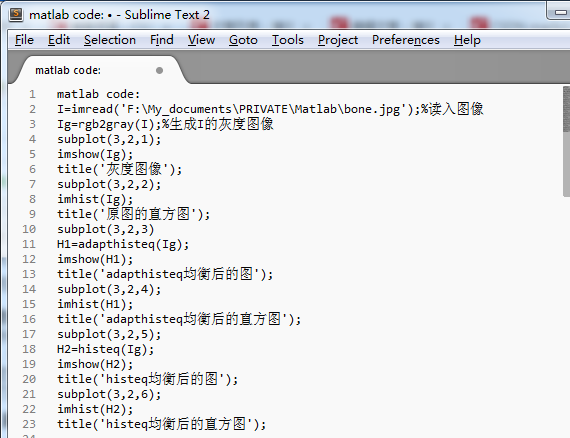效果图：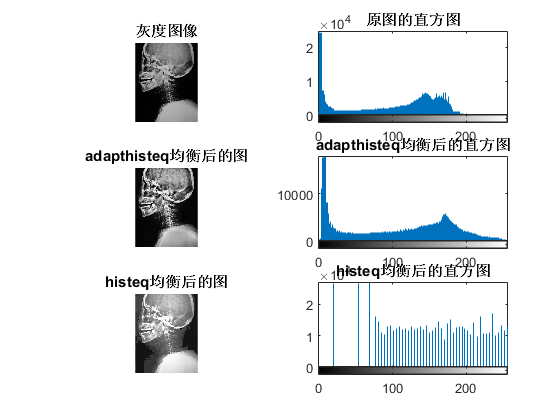由实验结果可知：

（1）   经过直方图均衡化后，图像的对比度明显增强了，视觉效果明显改善。

（2）   原始图像较暗且占据的灰度范围比较窄。均衡化后的图像的直方图的灰度值范围明显扩大。

（3）   经过histeq 均衡化后的图像的直方图趋于平坦，灰度级有所减少，某些灰度进行了合并。

2、直方图的匹配

直方图均衡化的优点在于能完全自动化，不需要人工参与，但其缺点是只能产生一种近似均衡的直方图。有时，人们希望修改一副图像的直方图，使得与给定的直方图匹配，或者具有一种预先的函数形状，也就要用直方图的匹配

例6：直方图的匹配过程可分为以下几步：

步骤：

1、求给定函数的累积直方图y;

2、求原图像的累积直方图J；

3、通过J=histeq(I,hgram) 求得匹配后的图像。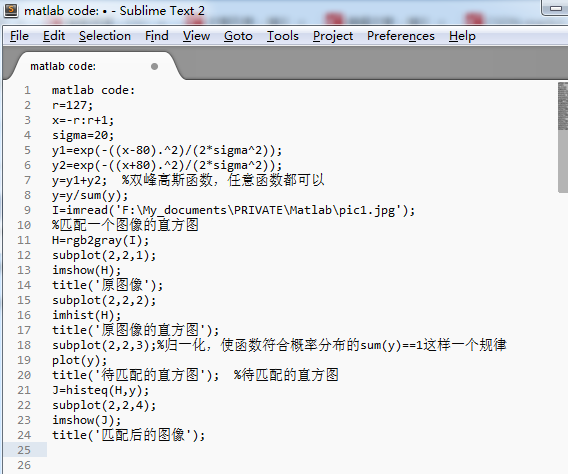效果图：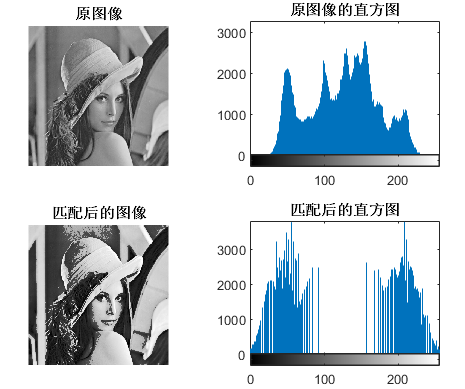展开全文图像预处理 图像增强 MATLAB
• 使用matlab 读取Lena.bmp图像，并将其显示为： 1、原始图像 2、左右翻转的图像 3、上下翻转的图像 4、放大一倍的图像 5、显示其直方图 6、图像向右下方平移半个图像长宽的距离 没有以分号结尾的话就会显示...
实验资源：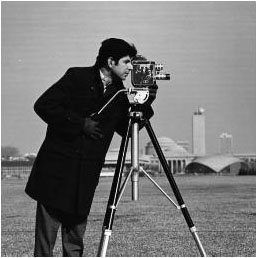实验要求：

使用matlab 读取Lena.bmp图像，并将其显示为：

1、原始图像

2、左右翻转的图像

3、上下翻转的图像

4、放大一倍的图像

5、显示其直方图

6、图像向右下方平移半个图像长宽的距离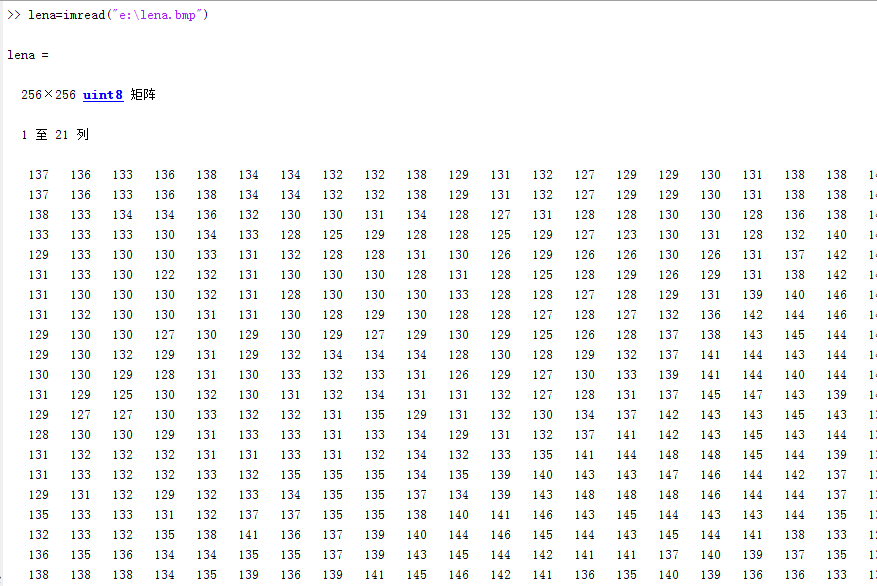没有以分号结尾的话就会显示出数据内容

读取图像并显示原始图像

imshow(lena);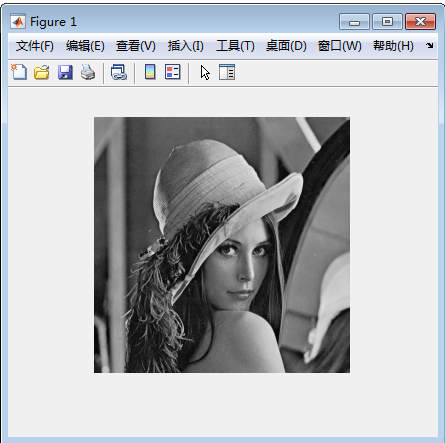左右翻转的图像

lena2=flip(lena,2);
imshow(lena2);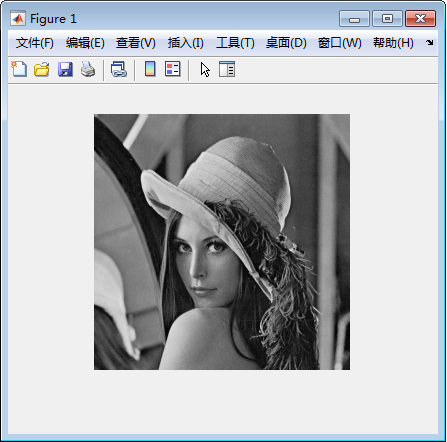上下翻转的图像

lena3=flip(lena);
imshow(lena3);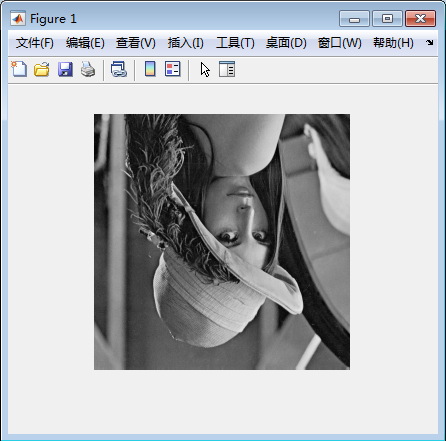放大一倍的图像

lena4=imresize(lena,2);
imshow(lena4);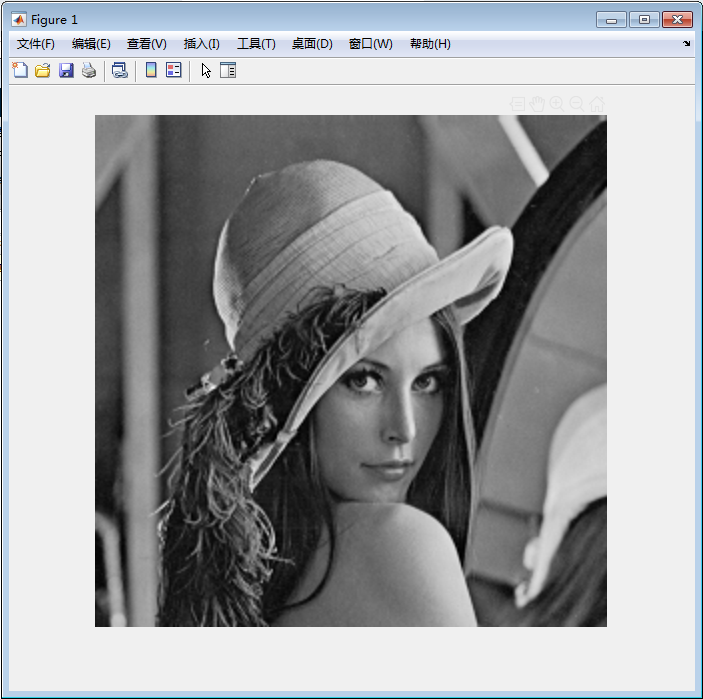显示其直方图

h=histogram(lena,255)
不以分号结尾。

这是lena的直方图：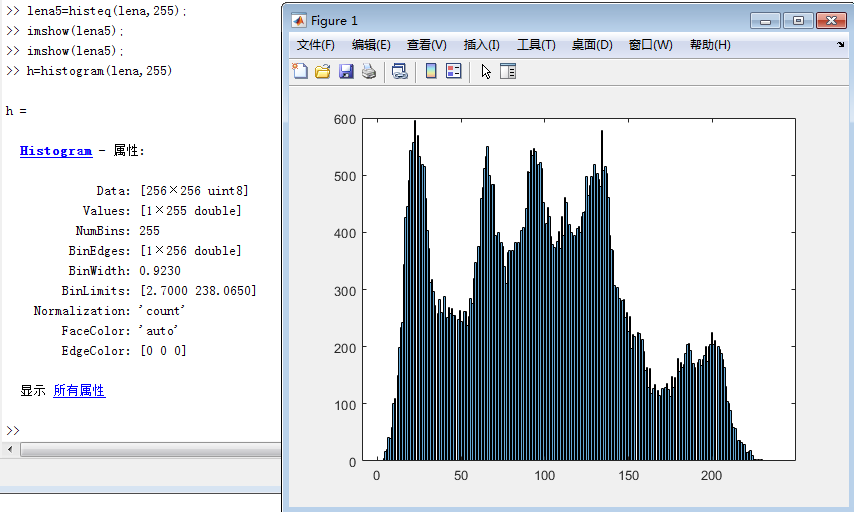imshow(lena5)显示的是一个比lena要更亮一点的图片（直方图均衡化）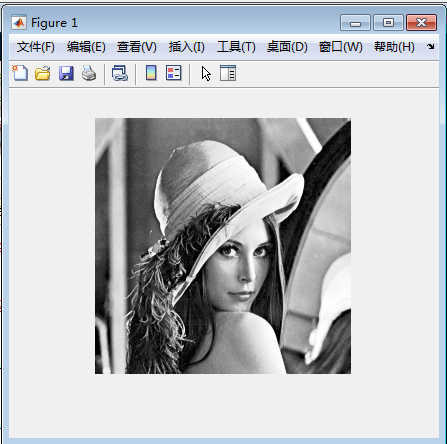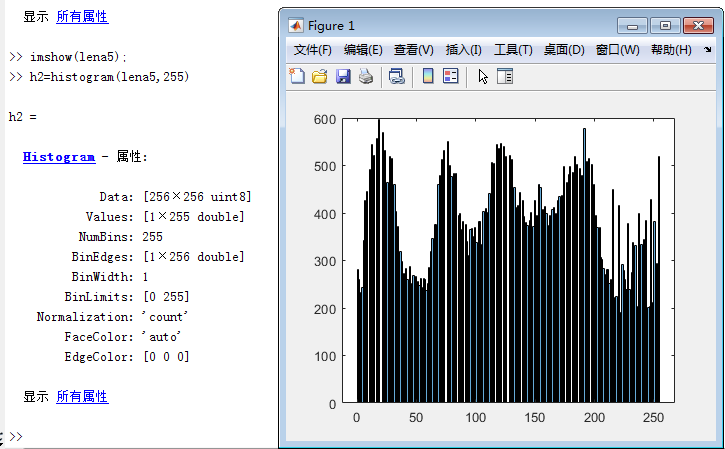图像向右下方平移半个图像长宽的距离

原理版：

% lena=imread("e:\lena.bmp");
[R, C] = size(lena); % 获取图像大小
res = zeros(R, C); % 构造结果矩阵。每个像素点默认初始化为0（黑色）
delX = 128; % 平移量X 原始图像的一半直接256/2指定128了，
% delX = R/2; % 平移量X
% delY = C*0.5; % 平移量Y，这些写法都可以
delY = 128; % 平移量Y
tras = [1 0 delX; 0 1 delY; 0 0 1]; % 平移的变换矩阵

for i = 1 : R
for j = 1 : C
temp = [i; j; 1];
temp = tras * temp; % 矩阵乘法
x = temp(1, 1);
y = temp(2, 1);
% 变换后的位置判断是否越界
if (x <= R) & (y <= C) & (x >= 1) & (y >= 1)
res(x, y) = lena(i, j);
end
end
end;

imshow(uint8(res)); % 显示图像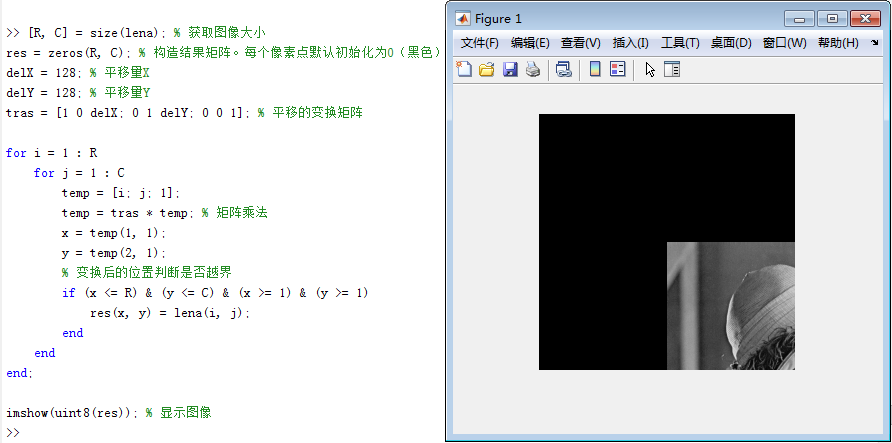函数版：

se=translate(strel(1),[128 128]);%构造结构元素
res=imdilate(lena,se);%形态学膨胀
figure;%显示图片框，以下内容都会显示在里面
subplot(1,2,1),subimage(lena);
title('orginal image');
subplot(1,2,2),subimage(res);
title('translate image');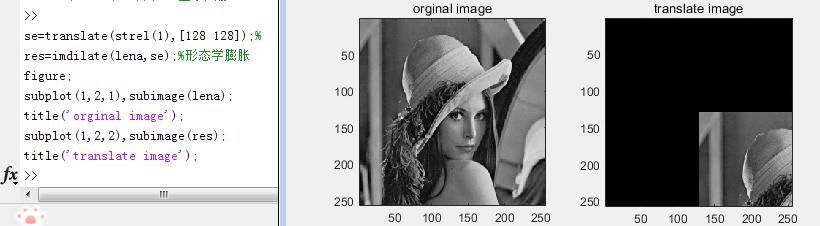figure;命令显示的变换后图片。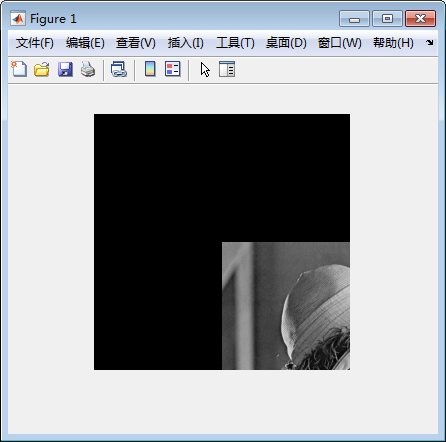这个版本的命令很简洁的样子，查阅一下其中的关键函数

translate  参考：https://ww2.mathworks.cn/help/matlab/ref/polyshape.translate.html

strel    参考：https://blog.csdn.net/qq_36792959/article/details/98979959

https://blog.csdn.net/qwerasdf_1_2/article/details/54376657

imdilate   参考：http://www.ece.northwestern.edu/support/local-apps/matlabhelp/toolbox/images/imdilate.html

https://www.mathworks.com/help/images/ref/imdilate.html

相关原理：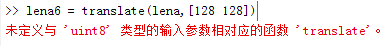当我们直接使用translate对lena进行操作时会报错，translate支持的是

输入 polyshape，指定为标量、向量、矩阵或多维数组。

数据类型： polyshape

strel(shape,parameters)。shape为形状参数，即设置什么样的结构元素；parameters为控制形状参数大小方向的参数。

SE =STREL('arbitrary',NHOOD)   创建一个指定邻域的平面结构化元素。NHOOD是一个包含1/0的矩阵；1的位置定义了邻域的形态学操作。NHOOD的中心就是它的中心元素，位置在FLOOR((SIZE(NHOOD) + 1)/2)。你也可以忽略参数串'arbitrary'而只使用STREL(NHOOD)。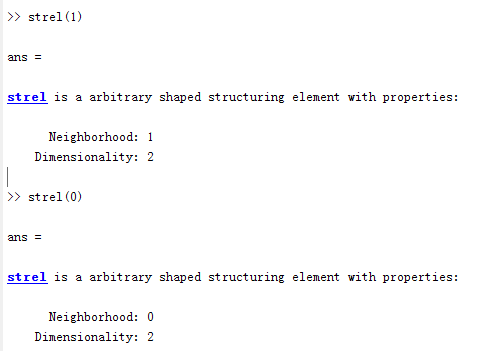随后实验证明strel（n）（n>1）都是和1一样的邻域大小

J = imdilate(I,SE)

扩大灰度，二进制或打包二进制（packed binary image）图像I，bmp是bitmap，返回扩大图像J。SE是由strel或offsetstrel函数返回的结构元素对象或结构元素对象的数组 。

J = imdilate(I,nhood)

扩展图像I，其中nhood是多个0和多个1 的矩阵，指定结构元素邻域。imdilate确定邻域的中心元素floor((size(nhood)+1)/2)。

这个语法相当于 imdilate(I,strel(nhood))

个人猜想：

以上思路就应当是想要使用translate 但是图像不是polyshape结构，所以先创造一个通用的结构元素（类似于java里的object），进行平移后再使用形态学函数填充结构元素。

在查阅上述原理的时候，由translate想到有没有支持通用图像的平移函数呢？毕竟是强大的matlab

果然找到了！

imtranslate

参考文档：https://www.mathworks.com/help/images/ref/imtranslate.html

一步到位版：

lena6 = imtranslate(lena,[128, 128],'FillValues',255);%底色填充为白色
lena6 = imtranslate(lena,[128, 128],'FillValues',0);%底色填充为黑色

>> [R, C] = size(lena); % 获取图像大小
>> lena6 = imtranslate(lena,[R*0.5,C*0.5],'FillValues',0);
>> imshow(lena6);

支持的输入参数（要转换的图像)

指定为任何类的非稀疏数值数组，除了uint64和int64，或逻辑数组。

数据类型：single | double| int8| int16| int32| uint8| uint16| uint32|logical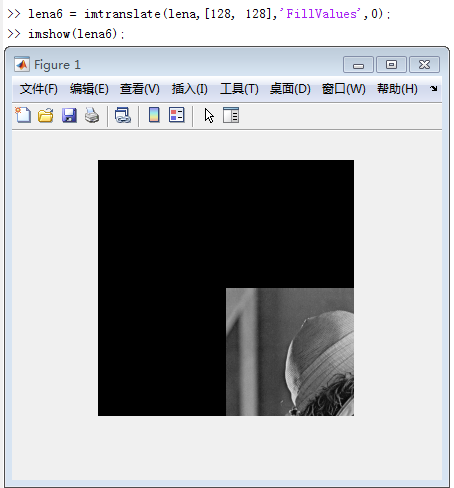简单而快乐~

展开全文• ## matlab里的图像处理函数

万次阅读 多人点赞 2019-02-19 15:56:54
一、imfinfo函数——查看图像文件信息，注意参数是文件路径和文件名，不是图像对应的矩阵。 &gt;&gt; imfinfo('C:\Users\zhulf\Desktop\1.jpg') ans =   Filename: 'C:\Users\zhulf\Desktop\1.jpg'  ...
一、imfinfo函数——查看图像文件信息，注意参数是文件路径和文件名，不是图像对应的矩阵。

>> imfinfo('C:\Users\zhulf\Desktop\1.jpg')

ans =
Filename: 'C:\Users\zhulf\Desktop\1.jpg'
FileModDate: '19-Apr-2015 08:40:54'
FileSize: 1528413
Format: 'jpg'
FormatVersion: ''
Width: 1548
Height: 1548
BitDepth: 24
ColorType: 'truecolor'
FormatSignature: ''
NumberOfSamples: 3
CodingMethod: 'Huffman'
CodingProcess: 'Sequential'
Comment: {}
Make: 'Smartisan'
Model: 'Smartisan T1'
Orientation: 0
DateTime: '2015:04:19 08:40:53'
DigitalCamera: [1x1 struct]
GPSInfo: [1x1 struct]
MeteringMode: 'unknown'
LightSource: 'unknown'
Flash: 16

二、imshow函数

1、imshow(I)  显示图像I

Matlab代码：

>> imshow(I)

显示结果2、imshow(I,[low high])  它显示的是像素处理后的图像I，注意的是它只是显示的时候改变了图像像素，实际上并没有改变图像像素，图像像素值还是原来的值。

Matlab代码：

>> imshow(I,[0 80])它对图像像素的处理是：将I中像素值大于等于high变成high，将小于等于low的变成low，再将[low,high]中间的像素映射为默认的像素级，一般为[0,255]。

我们把上述描述转为代码：

mmax=max(I(:));

mmin=min(I(:));

for i=1:256

for j=1:256

if I2(i,j)<=0 I2(i,j)=0;

elseif I2(i,j)>=80

I2(i,j)=255;

end

end

end

I3=I2;

for i=1:256

for j=1:256

I3(i,j)=255/80*I3(i,j);

end

end

imshow(I3)

显示图像结果为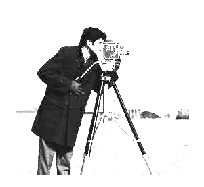和imshow(I,[0 80])效果是一样的。

3、 imshow(I,[ ])  它等同于imshow(I,[min(I:),max(I:)])，可以理解为把图像I转化成0-255灰度级来显示。

我们在这里做一个实验：下面三幅图分别为imshow(I)，imshow(I,[])和把图像像素值映射为[0,255]所显示的图像。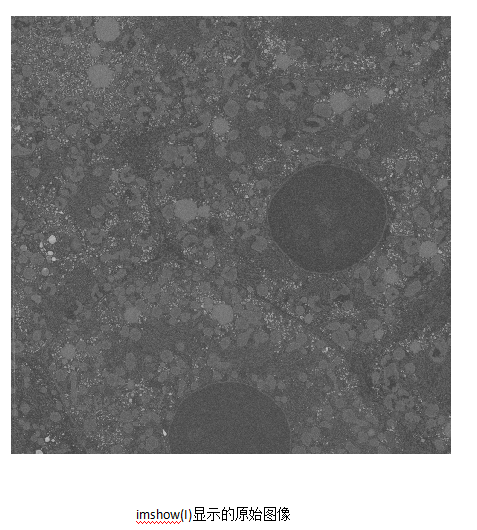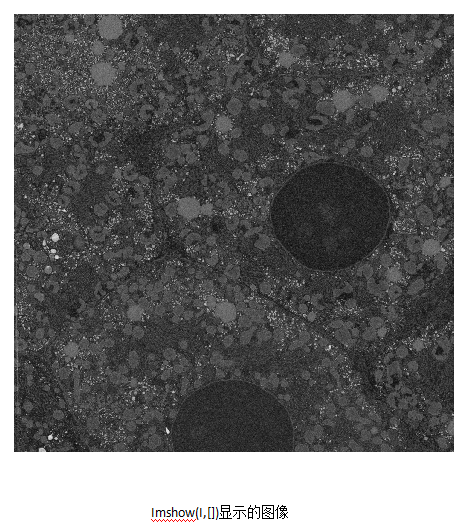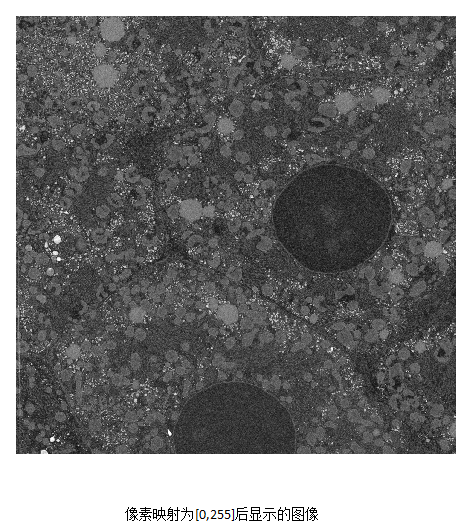我们发现，imshow(i,[])显示的效果正和把像素值映射为[0,255]效果所显示的一样。

Matlab代码如下：

mmax=max(A(:));

mmin=min(A(:));

for i=1:4096

for j=1:4096

A2(i,j)=1.0*255/(mmax-mmin)*(A(i,j)-mmin);

end

end

imshow(A)

figure,imshow(A,[])

figure,imshow(A2)

再例如，一个灰度级为17-2424的int16类型的图像，matlab显示(imshow(I))效果如下，可见，显示效果并不好，接下来我们使用imshow(I,[])，显示结果如下：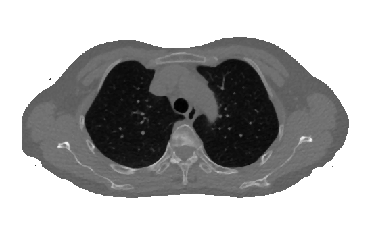显示结果较好，对比度较高。同样，imshow(I,[])的效果等同于把像素映射到0-255灰度级，映射后图像如下：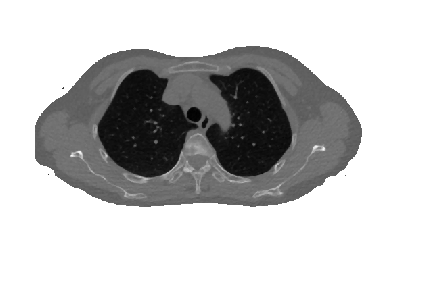实现灰度映射的Matlab如下：

mmax=double(max(A(:)));  %类型转换很重要

mmin=double(min(A(:)));  %类型转换很重要

for i=1:512

for j=1:512

tmp=double(A(i,j));  %类型转换很重要

A2(i,j)=uint8(255*(tmp-17)/2407);  %类型转换很重要

end

end

imshow(A)

figure,imshow(A,[])

figure,imshow(A2)

上面是对imshow()函数的理解，不明白的欢迎留言交流。

三、rgb2gray 将彩色图像转换为灰度图像

>> B=rgb2gray(A);
>> imshow(A)

>> figure,imshow(B)

四、数据类型转换函数

im2uint8  将图像转换为uint8类，相应的像素值也会变化到相应范围，如对uint16类图像应用im2uint8，则像素值由0-65535变化成0-255，这是一种线性映射。

im2uint16 将图像转换为uint16类，也有上面如此的性质。

im2double 将图像转化为double类，必要时缩放像素值。如果图像img是double型的，d=img;如果图像是logical或single型图像，d=double(img);如果图像是uint8型，d=double(img)/255；如果图像是uint16型，d=double(img)/65535。

im2double一般会将uint8归一化到[0,1],而double只是将数据变为double,如：

a = uint8(250);
b = double(a)
c = im2double(a)
b =
250
c =
0.9804

im2single 将图像转化为single类，性质和im2single类似。

mat2gray 可以将图像转换为标定[0,1]范围的double类的图像。

调用的语法是：

g=mat2gray(A,[Amin,Amax])，其中g具有0（黑）到1（白）的值。参数Amin使得A中小于Amin的值，在g中变为0；而在A中大于Amax的值，在g中变为1。

也可以是:

g=mat2gray(A)，

Amin,Amax默认为A中实际的最大值和最小值，它独立于输入的类，将整个输入值的范围标定为[0,1]。

>> f=[1 2;3 4]
f =
1     2
3     4
>> g=mat2gray(f)
g =
0    0.3333
0.6667    1.0000

BW = im2bw(I,LEVEL)   二值化图像，将大于LEVEL的像素值变为1，小于LEVEL的像素值变为0，且图像像素变成逻辑类；LEVEL是阈值，在[0,1]之间。

>> gb=im2bw(g,0.6)
gb =
0     0
1     1

>> gbv=islogical(gb)
gbv =
1

五、whos

B为图像矩阵

>> whos B
Name         Size                Bytes  Class    Attributes
B         1548x1548            2396304  uint8

六、反转图像

>> imshow(I)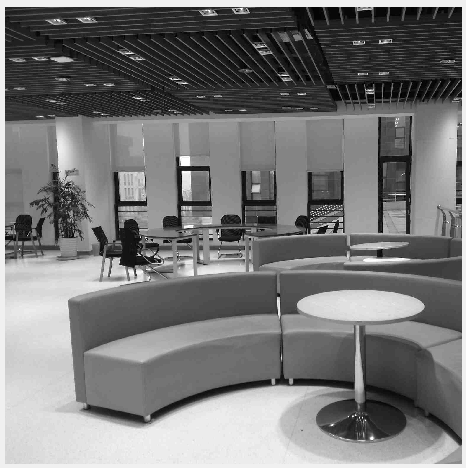上下翻转：

>>I2=I(end:-1:1,:);

>> figure,imshow(I2)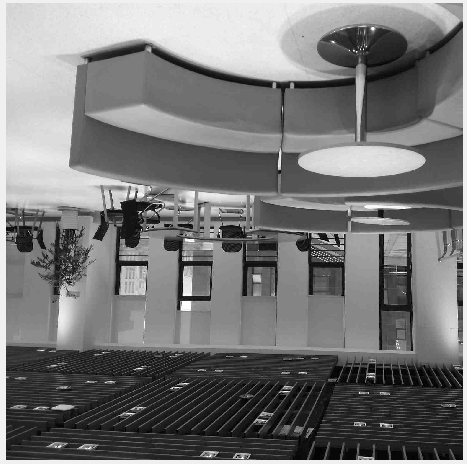上下左右均反转：

>> I3=I(end:-1:1,end:-1:1);
>> figure,imshow(I3)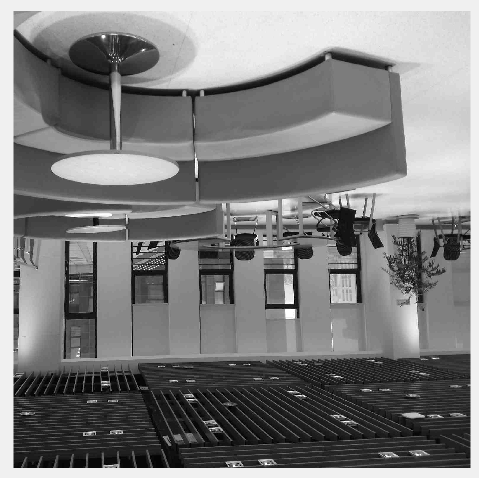七、抽取图像（压缩图像）

>> I4=I(520:855,90:837);
>> figure,imshow(I4)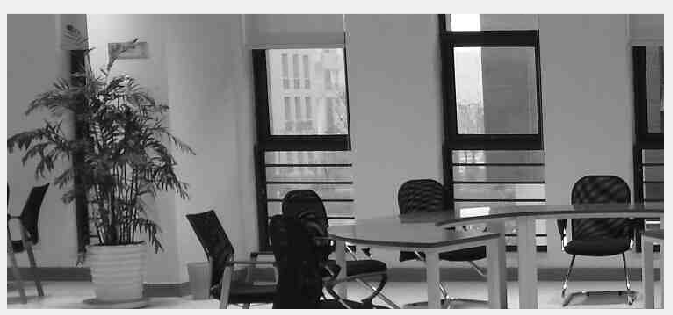图像也由原来的1548 x 1548变成了336 x 748。

>> I5=I(1:2:end,1:2:end);
>> figure,imshow(I5)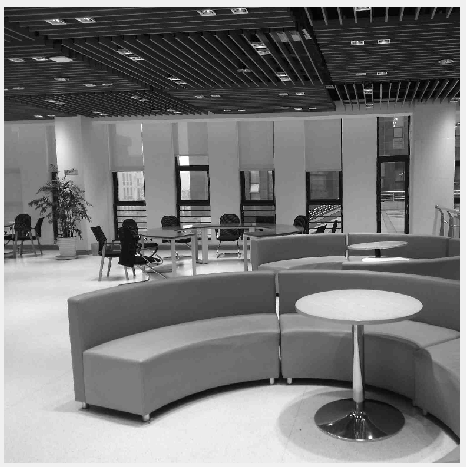图像由1548 x 1548 变成了774 x 774，filesize由99745变成了76747，做了部分压缩，但清晰度差不多。

八、improd()函数

function[p,pmax,pmin,pn]=improd(f,g)
%IMPROD Computes the produce of two images.
%[P,PMAX,PMIN,PN]=IMPROD(F,G) outputs the element-by-element
%product of two input images,F and G,the product
%maximum and minimum values,and a normalized product array with
%values in the rang[0,1].The input images must be of the same
%size.They can be of class uint8,uint16,or double. The outputs
%are of class double.
fd=double(f);
gd=double(g);
p=fd.*gd;
pmax=max(p(:));
pmin=min(p(:));
pn=mat2gray(p);

>> [p,pmax,pmin,pn]=improd(f,g)
p =
1     4
6     4
pmax =
6
pmin =
1
pn =
0    0.6000
1.0000    0.6000

之后陆续补充。。
展开全文imshow matlab 图像处理
• 用函数对cameraman图像进行非线性变换 a=imread(‘F:\work\Digital Image Processing\2015\ketangshiyan\ceshitu\cameraman.jpg’) ; %读取原始图像 figure(1); imshow(a); xlabel(‘(a)原始图像’); x=1:225; ...图像处理 matlab
• 为一幅图片添加椒盐噪声，高斯噪声，然后用均值滤波和中值滤波，进行滤波处理这两种噪声，比较两种滤波方式。 实验步骤 详见代码 实验结果 添加噪声 滤波结果 从实验结果看，对于椒盐噪声中值滤波...图像处理 Matlab
• 第五章图像增强 两个方法： 1）空间域增强：点处理，模板处理即领域处理 2）频率域增强：高低通滤波，同态滤波 1.空间域图像增强 1）点处理 基于灰度变换 灰度线性变换 作用：对每一个像素灰度...matlab 知识点总结 数字图像处理
• 下面简单介绍一下MATLAB中的图像处理过程： 1、打开MATLAB主界面后，在编辑器中写入以下代码： I=imread('G:\MATLAB\bm.bmp'); J=double(I); J=-J+(256-1); %图像反转线性变换 H=uint8(J); subplot(1,2,1),...MATLAB 图像处理
• ## 图像处理（matlab）

万次阅读 2018-09-11 22:35:04
1.基本函数: iread('文件路径'); idisp(); 2.一元操作： imd=idouble(im);... //将彩色图像转变成灰度图像。 ihist（）；//显示每一个像素值出现的次数 im=igamma(im,1/0.45); //伽马编码，一种非线性一...
• ## MATLAB 编写GUI进行图像处理

万次阅读 多人点赞 2018-06-29 10:14:03
GUI，即图形用户接口，是MATLAB的可视化操作功能，本文编写一个简单GUI进行图像处理。1、新建GUI文件，点击file-&gt;new-&gt;GUI如下图所示点击GUI后出现如下图界面，默认为Blank GUI (Default)选项，点击OK...MATLAB 图像处理 GUI
• 本书主要介绍MATLAB语言在图像处理技术方面的应用，重点介绍了各种图像处理方法的理论和实际应用，书中给出了大量MATLAB实例和图像处理效果，使渎职能够更好的理解和掌握使用MATLAB图像处理工具箱函数进行图像处理的...
• 写在前头：说到数字图像处理，不得不提起MATLAB。这是一款非常方便的仿真软件，绝大多数的图像处理可以用MATLAB完成。 　有人问，处理图片，用PS岂不是更好。两者各有优点，如果需要将1００００幅图片转换成灰度...图像处理 matlab 图片
• 计算机图像处理实验课1-9，看完本课程可以让你matlab技能小小进步，对图像处理稍微了解。、 （一）matlab数字图像处理实验-guide的简单使用教程 （二）matlab数字图像处理实验-图像的几何变换 （三）matlab数字...matlab 图像处理
• Matlab计算机视觉/图像处理工具箱推荐 2014年4月9日机器学习MATLAB, 计算机视觉luffylee 计算机视觉/图像处理研究中经常要用到Matlab，虽然其自带了图像处理和计算机视觉的许多功能，但是术业有专攻，在进行...
• 图像处理matlabOpenCV基本操作 图像的读写 matlab读取和保存图像 OpenCV读取和保存图像图像处理matlab+OpenCV基本操作 引言 本文记录常用的图像操作在matlab和OpenCV中的接，持续更新（2016.5.28） 图像的读写...opencv matlab
• MATLAB具有丰富的图像处理函数库，运算速度慢，特别是在多重循环的情况下，不适合直接应用于工程当中。如果能把MATLAB和另一种适合工程的编程语言结合到一起运用到数字图像处理领域，则会更加方便的进行图像处理，...
• ## 数字图像处理——基于Matlab

万次阅读 多人点赞 2020-06-28 10:54:53
（注：本文代码大部分可从《数字图像处理 第三版》中找到）使用软件：MATLAB R2018a学习前提：了解matlab的GUI界面的每个按钮参考资料：《数字图像处理 第三版》，CSDN博客使用初音图片P站画师uid：1589657。...matlab 数字图像处理 GUI 学习笔记
• 今天发现了matlab图像处理工具箱的强大之处， 任务缘由： 需要对图像进行批处理改变尺寸 一般遇到这种任务，我们往往都会写matlab代码进行批量操作 但是matlab有自带的很强大的图像处理工具箱，  图片批处理...matlab图像处理
• 图像旋转 图像的旋转其实矩阵的旋转，而整个矩阵的旋转，则可以看出单个坐标的旋转。也就是说，只有我们知道了单个坐标旋转后的坐标，那么就很好得出旋转之后的图像了。这里我们假定旋转后的图像大小不变哈。 对于看...MATLAB 数字图像处理 图像旋转
• 由于这段时间在一个图像处理与三维重建的项目，其中数字图像处理的知识是必不可少的，学习matlab也有一段时间了，所以现在抽点时间将这段时间所学的东西个小总结，加深理解的同时也方便以后有需要时可以查看一下...图像处理 matlab
...